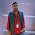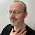Sunday, 22 July 2012

Introduction to C language bitwise operators: " _BV " Macro (...cont)

```
```
```
```
```/* The  _BV() is a compiler macro defined as #define _BV( bit ) ( 1<<(bit) ) in
<avr/sfr_defs.h> which was included already indirectly through <avr/io.h>.
It stands for Bit Value where you pass it a bit and it gives you the byte value
with that bit set.
As we seen already, in the C language one assigns and tests bits using bit
operators, the assign operator, and the concept of bit masks: */```
```PORTC |= 0x01;  // Set bit 0 only.
PORTC &= ~0x01; // Clear bit 0 only.
PORTC ^= 0x01;  // Toggle bit 0 only.
PORTC & 0x01;  // Test bit 0 only.
PORTC |= 0x80; // Set bit 7 only.

/*Using macros make this easier to read. The _BV() macro in avr-libc takes a number as   the argument and converts it to the appropriate bit mask. (The BV stands for Bit Value).   The _BV() macro is defined as: */```
`#define _BV(x)   (1 << x) `
```/* This allows */
PORTC |= _BV(0);  // Set bit 0 only.
PORTC &= ~(_BV(1));  // Clear bit 1 only.
PORTC ^= _BV(7);  // Toggle bit 7 only.

/*Using bit operators, one can do multiple, non-contiguous bits at a time: */

PORTC |= (_BV(0) | _BV(2) | _BV(7));  // Set bits 0,2,7
PORTC &= ~(_BV(1) | _BV(2) | _BV(6));  // Clear bits 1,2,6
PORTC ^= (_BV(5) | _BV(3));   // Toggle bits 3,5

/*The | symbol between each _BV macro statement means logically OR. */
/*(_BV(0) | _BV(2) | _BV(7));  logically OR’s the bits together
e.g*/
/* Name        bit7  bit6  bit5  bit4  bit3  bit2  bit1   bit0
_BV(0)  =    0      0    0     0      0     0    0      1
_BV(2)  =    0      0    0     0      0     1    0      0
_BV(7)  =    1      0    0     0      0     0    0      0
or’ed   =    1      0     0     0     0     1     0      1
*/

/*   A further example is */```
```UCSRB = _BV(TXEN)|_BV(RXEN)|_BV(RXCIE); /* tx/rx enable, rx complete*/

/*  In each iteration of the infinate loop (while (1)) we are using   standard C methods for setting and clearing the PC0 bit followed by   1000 mS delay between each set and each clear. Let's take a closer   look at each of those.   The first, is to clear the bit using PORTC &= ~_BV(PB0);   which turns off the PIN (remember that the PIN is connected to   VCC, so a logical 1 output results in little to no   voltage across the PIN).   Remember that our _BV macro returns the bit set in byte form,   in this case, 0x01 or 00000001b. So, the statement PORTB &= ~_BV(PB0);  is actually PORTB &= ~0x01;.   The bitwise operator ~ will "not" the value first, which results   in 11111110b. So now we basically have PORTB &= 0xFE;. With this,   the bit in position 0, PB0, will ALWAYS be cleared after this   statement without effecting the other bits in the byte. So   regardless of the value currently in PORTB, only the bit we're   clearing is changed. */

```
```#define F_CPU 16000000UL /* 16 MHz Internal Oscillator */

#include <avr/io.h>
#include <util/delay.h>

/* function for long delay */
void delay_ms(uint16_t ms) {
while ( ms )
{
_delay_ms(1);
ms--;
}
}

int main (void)
{
/* PB0 is digital output */
DDRB = _BV (0);

/* loop forever */
while (1)
{
/* clear PB0 on PORTB (digital high) and delay for 1 Second */
PORTB &= ~_BV(0);
delay_ms(1000);

/* set PB0 on PORTB (digital low) and delay for 1 Second */
PORTB |= _BV(0);
delay_ms(1000);
}
}
```

1.Thank you for the post!

2.3.Thank you very much ! Great !

4.版主
我最近在做4*4*4的LED
但使用PIC18F4525
但矩陣寫太多就會錯誤
我只到8BIT 只能到255的矩陣
但是想要讓LED能有多種變化
不知道有否其他寫法
寫在DATA BUS 的方式有嗎??

5.In the 'Clear' operations &= can you explain why a tilde ~ is needed before the bit is specified? Why is the tilde not used in any of the Set, Toggle or Test operations?

1.the "~" (tilde) character means something like "take any 1 bit and make it 0, take any 0 bit and make it 1". As we are &'ing it with the Bit 0, we basically filliping it alone, as we are using the BV macro. Your latter question is a good one... Been a while since i used AVR.

6.I have been searching for a long time for this information. Every where else they seem to copy what someone else has written and they make a grave error. They say that the _BV command shifts a value to a location. If it "shifts" then why don't the other bits change? Errors in how things are stated cause a lot of confusion for the new users. I had finally determined that the "_BV" command sets a bit but wasn't sure till I read your post. I thank you for your time you spent making things clear.

7.I have a question in this line (PORTC |= 0x80; // Set bit 7 only.).
I don't understand why this code can control bit 7. I think bit 7 in decimal is going to be 64 in decimal which means it's going to be 0x40 in hex. In this case setting bit 7 is going to be (PORTC |= 0x40;) isn't it ?

1.When counting bits you start from 0, meaning that bit 7 refers to the bit in the 8th position.

0x80 -> 10000000
0x40 -> 01000000

Feel free to contact me with any suggestions, doubts or requests.

Bless